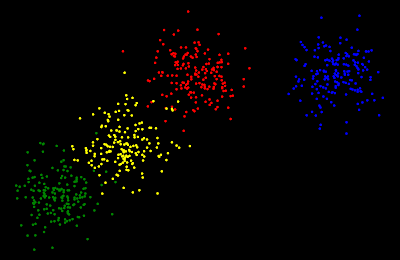### Implementing Hierarchical Clustering - In Python Programming language

Introduction to Hierarchical Clustering

Unsupervised learning is a type of Machine learning in which we use unlabeled data and we try to find a pattern among the data.
Clustering algorithms falls under the category of unsupervised learning. In these algorithms, we try to make different clusters among the data.
Hierarchical Clustering algorithms build a hierarchy of clusters where each node is a cluster consisting of the clusters of its children node.fig. 1

Check out my blog on Hierarchical Clustering - An Unsupervised learning Algorithm to learn more about it.

Implementing it with Python and SciKit-Learn

We will use the python programming language for its implementation. In python language, we will be using SciKit-Learn library. So let's start with implementation -
We will be using our own dataset which will be generated by us.

1. Importing necessary Libraries -
To create a machine learning model we will use SciKit-Learn (sklearn) library and to create our dataset we will use SciPy library.

2. Creating our dataset and Plotting it
The dataset which we created and plotted will look something like this 👇

To play with the dataset you can change the parameters of make_blobs method. If you need to increase more examples then increase the value of n_samples, you can also change centres of clusters and distance between the clusters.

3. Preprocessing dataset and Training a Model

5. Plotting the generated clusters

The output of the above code is as follow -
The red and green clusters are a little bit off but you can try to improve it.

6. Creating Dendrogram

The dendrogram will look like -

1.